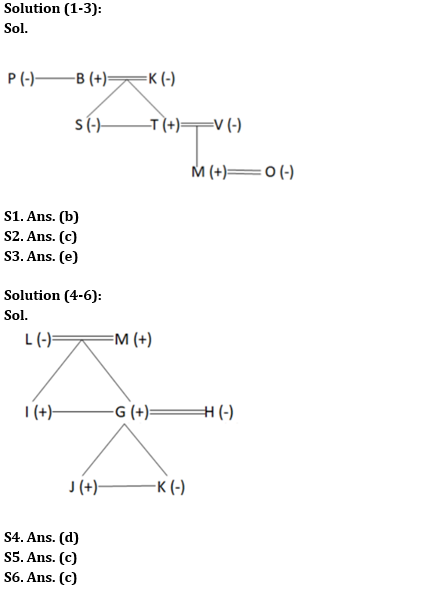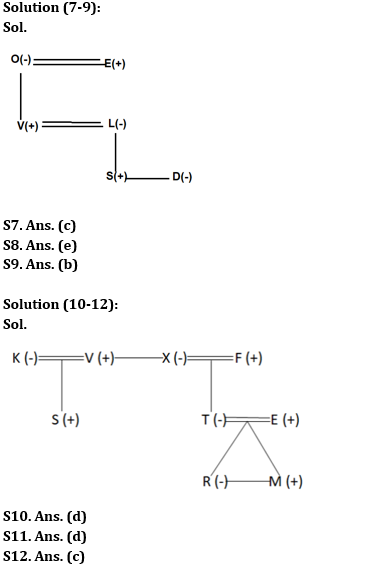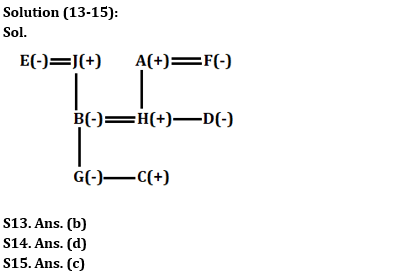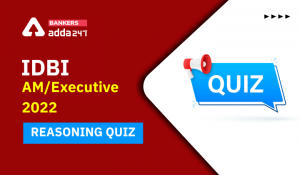Latest Banking jobs   »

# Reasoning Ability Quiz For IBPS RRB PO Prelims 2022- 30th May

Directions (1-3): Study the information carefully and answer the questions accordingly.
There are eight members in a family with three married couples. P is the aunt of T who is the son of K. O is the daughter-in-law of V. B is the father of T and S who is unmarried. S is the sister-in-law of V. M is the grandson of K who does not have a sibling. P has no sisters.

Q1. Who is the husband of O?
(a) K
(b) M
(c) T
(d) B
(e) None of these

Q2. How M is related to B?
(a) Uncle
(b) Son
(c) Grandson
(d) Nephew
(e) None of these

Q3. Four from the following five are similar in a certain way and thus form a group. Find out the one who does not belong to that group?
(a) P
(b) K
(c) S
(d) O
(e) T

Directions (4-6): Study the information carefully and answer the questions accordingly.
There are seven members in a family. H is the daughter-in-law of L who is the grandmother of K’s brother. M is the father of two children in which one of them is married. G and H are married to each other. K is the sister of J. J is the only son of G whose brother is I.

Q4. How M is related to G?
(a) Brother
(b) Son
(c) Grandfather
(d) Father
(e) None of these

Q5. Who is the father of K?
(a) L
(b) M
(c) G
(d) I
(e) None of these

Q6. How many female members in the family?
(a) One
(b) Two
(c) Three
(d) None
(e) More than three

Directions (7-9): Study the information carefully and answer the questions accordingly.
S, O, L, V, E, and D are six members in a family of three generations. There are two married couples among them. L is the mother of S and D. E is the father of V. S is the grandson of O. The total number of female members in the family is three. V is a married person with two children

Q7. Which of the following pair is one of the married couples?
(a) E-D
(b) O-V
(c) E-O
(d) S-D
(e) None of these

Q8. How is D related to S?
(a) Brother
(b) Daughter
(c) Son
(d) Mother
(e) None of these

Q9. Which of the following is a group of male members?
(a) OVE
(b) SVE
(c) VLD
(d) OLD
(e) None of these

Directions (10-12): Study the information carefully and answer the questions accordingly.
T is the only child of F. F has no sister. E is the son-in-law of X. V is the brother-in-law of F. X does not have a niece. K and V is a couple. S is the cousin of T. R is the granddaughter of X. M is the son of T. X is the wife of F.

Q10. How V is related to T?
(a) Niece
(b) Nephew
(c) Father
(d) Uncle
(E) None of these

Q11. How many female members are there?
(a) Three
(b) One
(c) Two
(d) Four
(e) Can’t be determined

Q12. How F is related to M?
(a) Nephew
(b) Uncle
(c) Grandfather
(d) Father
(e) None of these

Directions (13-15): Study the information carefully and answer the questions accordingly.
A three-generation family consists of nine members i.e. A, B, C, D, E, F, G, H and J where number of females are more than the number of males. D is the sister of H. B is the mother of G. J is the grandfather of G. F is the mother of H. A is the father-in-law of B. C is the sibling of G. A’s spouse have only two children. Cis the grandson of H’s mother-in-law.

Q13. What is the relation of F with respect to B?
(a) Maternal Aunt
(b) Mother-in-law
(c) Father
(d) Daughter-in-law
(e) Maternal Uncle

Q14. If N is the husband of G, then what is the relation of N with respect to H?
(a) Son
(b) Father-in-law
(c) Brother-in-law
(d) Son-in-law
(e) None of these

Q15. Which of the following is true with respect to the given arrangement?
(a) F is the father-in-law of D
(b) D and H are sisters.
(c) Only five female members are there in the family.
(d) J has two granddaughters.
(e) All are true

Solutions#### Congratulations!Incorrect details? Fill the form again here

•Reasoning Ability Quiz For IBPS RRB PO P...
•Reasoning Ability Quiz For IBPS RRB PO P...
•Reasoning Ability Quiz For IDBI AM/Execu...
•Reasoning Ability Quiz For IBPS RRB PO P...
•Reasoning Ability Quiz For IBPS RRB PO P...
•Reasoning Ability Quiz For IDBI AM/Execu...